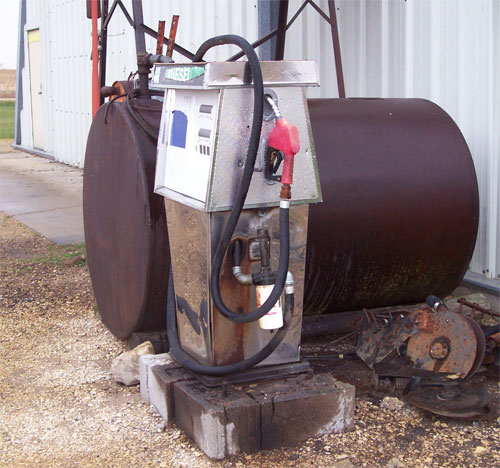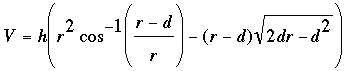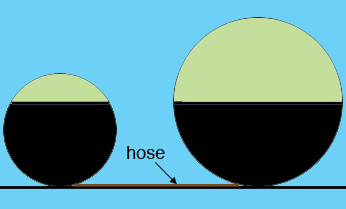SEARCH HOMEMath Central Quandaries & QueriesQuestion from Dave: How many gallons are left in a 36x60 in. barrel (laying on its side) and has 16 in. of gasoline left. I have attached a diagram.Dave,The volume of a cylinder is V = π r2 h where π is approximately 3.14159, r is the radius of the circular end and h is the height if the cylinder is standing on end. For your barrel r = 36/2 = 18 inches and h = 60 inches. Thus the volume is V = 61072.56 cubic inches. There are 231 cubic inches in a US gallon so the volume is V = 61072.56/231 = 264.4 gallons.

The expression for the volume of fuel remaining in the tank is given in a response to a previous question. I have copied it below.

Here d is the depth of the fuel. Since your measurements are in inches this expression will give the volume in cubic inches. To use this expression you must have your calculator set to calculate cos-1 in radians not degrees.

You have d = 16 inches so the expression yields 26225.18 cubic inches which is 26225.18/231 = 113.5 gallons. Hence the space remaining in the barrel in gallons is 264.4 - 113.5 which rounds to 151 gallons.

Harley

In December of 2013 Dennis Warwick from West Carolina Water Treatment sent us an Excel spreadsheet that implements the formula above. Dennis has agreed to allow Math Central users to download and use his spreadsheet. The default units are inches and gallons but if you click on "in" or "gal" you will be presented with a pull down menu of other options.

Thanks Dennis,

Harley

In Jauary 2014 Jonathan sent the following question.

Question from Jonathan:

I have found a partial answer to my question here on this page but I have two barrels on there side connected together with a hose at the bottom (lowest point).

How would the formula be altered to reflect this?
My situation is an oil storage rack with 2 drums linked that we need to inventory.

Thanks so much for your help

Hi Jonathan,

I drew a sketch of what I think you are describing. If I am correct then the level of oil is the same in the two tanks. You can use that level and the dimensions of the tanks with Denis' spreadsheet to find the amount of oil in each tank.HarleyMath Central is supported by the University of Regina and The Pacific Institute for the Mathematical Sciences.